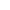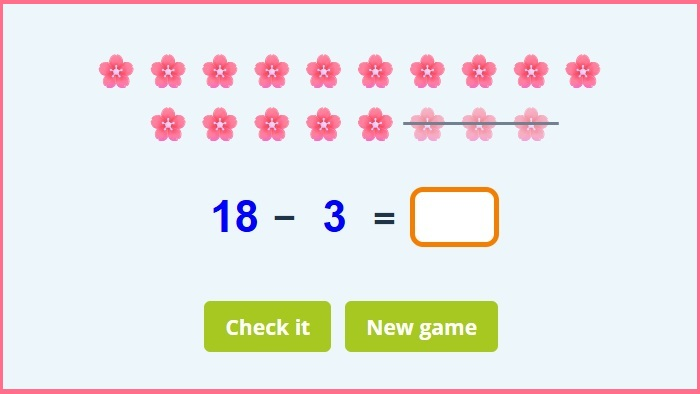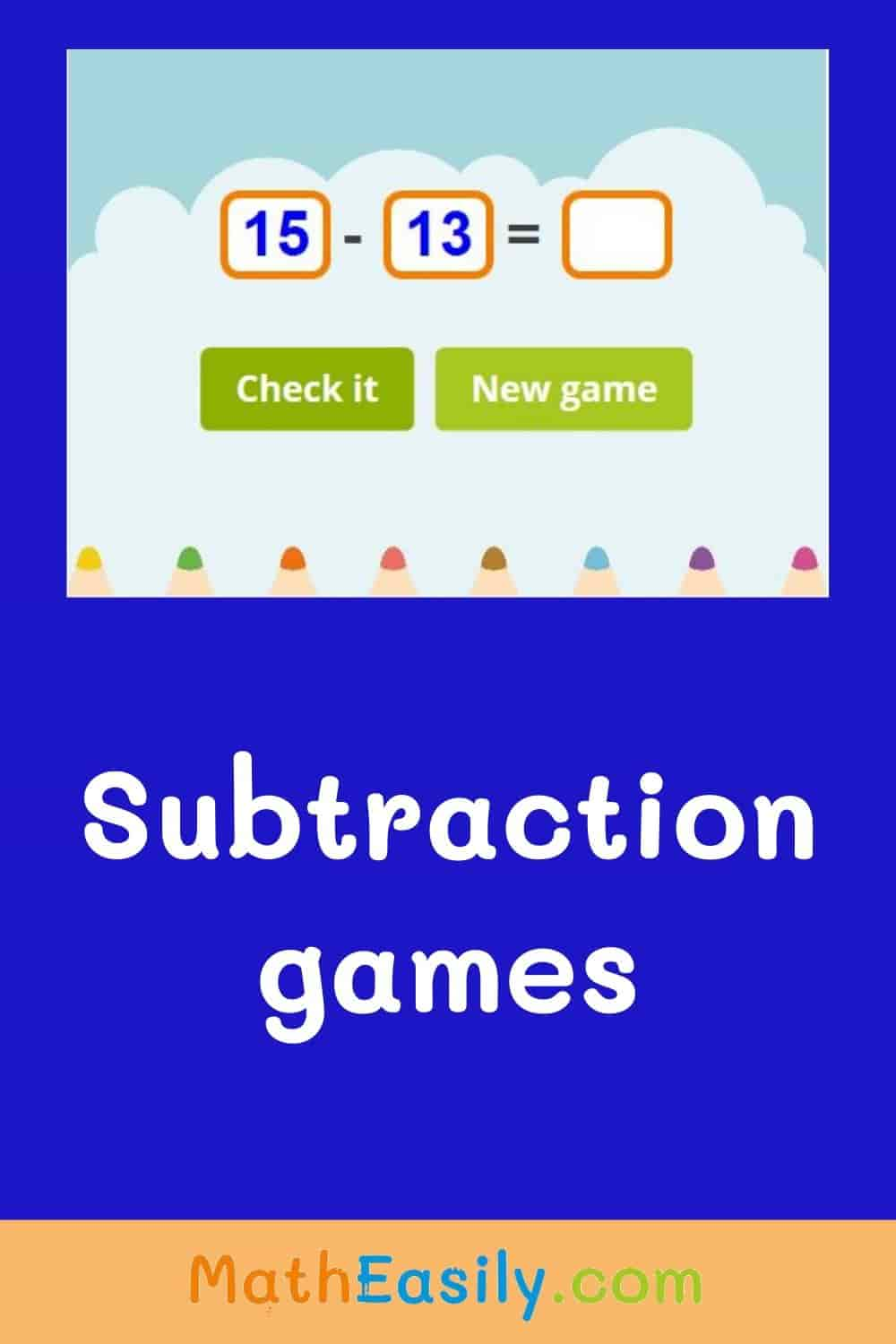﻿ Picture subtraction within 20 games + worksheets

# Subtraction within 20 games + worksheets

Addition and subtraction within 20 games online. Subtraction using pictures up to 20. Subtraction within 20 worksheets. Subtraction up to 20 with pictures. Picture subtraction worksheets to 20. Subtraction with pictures up to 20. math subtraction pictures.

New gameFill in the result of picture subtraction to the empty box.

## How to solve this subtraction within 20 with pictures?Insert the result of the subtraction to the empty box. Press "Check it" to find out if your answer is correct. If your result of subtraction is OK, the box will be green. If your answer isn´t correct, the box will be red and you will see the correct number.

Press the button "New game" and play another subtraction with pictures up to 20 game.

Nice pictures help kids to solve subtraction to 20 game more easily. The total amount of pictures represents the largest number. From this number kids remove crossed out pictures. What remains is the right answer.

You can also learn to subtract within 20 with these subtraction puzzles. Uncover the hidden picture.

## Subtraction within 20 games online

You can play subtraction within 20 games online as many times as you want or you can download free picture subtraction worksheets.

To play subtraction within 20 games kids already should know how to subtract within 10.

Do you want to try something more difficult? Then play subtraction within 100 game.

Do you want to practice addition and subtraction at the same time? Then play this math crossword puzzle game.

Watch this nice tutorial on YouTube which teaches kids basics of subtraction.## Picture subtraction problems

This online subtraction game teaches kids to subtract numbers up to 20.

Subtraction using pictures for Grade 1 is one of our most popular free online math games for grade 1.

More than 400 picture subtraction problems available.